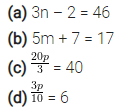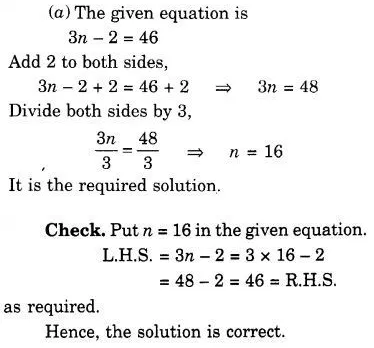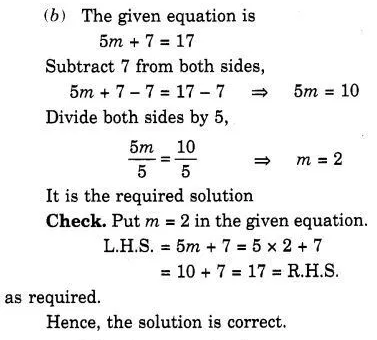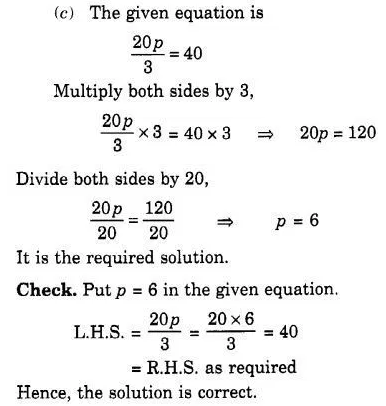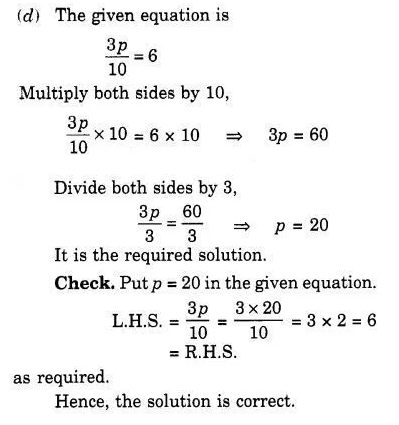# Give the steps you will use to separate the variable and then solve the equation : (a) 3n – 2 = 46

Give the steps you will use to separate the variable and then solve the equation :